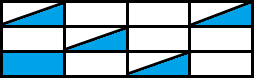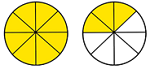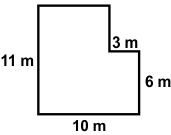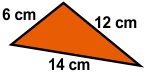# CREST Mathematics Olympiad Class 4 Previous Year Papers

## Syllabus:

Section 1: Numerals and Number Names, Number Sense (5-digit numbers), Computation Operations, Fractions, Length, Weight, Capacity, Time, Money, Geometry, Perimeter of Various Shapes, Symmetry, Conversions, Data Handling.

Achievers Section: Higher Order Thinking Questions - Syllabus as per Section 1

 Q.1 Seren bought 5 kg of fruits. His neighbour borrowed three-fifth of the fruits from him. What amount of fruits was borrowed?
 Q.2 What fraction of the figure is shaded ?Q.3 A jug can hold five times the quantity of water that a glass can hold. If the jug holds 3 L of water, what is the capacity of glass?
 Q.4 Amy's monthly income is 36,000 cents. Two-third of it is used for paying house rent and one-fourth is used for household expenditure. How much money is left with him?
 Q.5 Mitti bought 5 stick ice-creams, 4 cone ice-creams, and 6 cup ice-creams. The cost of a stick ice-cream, a cone ice-cream, and a cup ice-cream is 35 cents, 45 cents and 30 cents, respectively. Find the total amount of money paid by her:
 Q.6 Express the following picture as mixed fraction:Q.7 What number will come at the hundred's place if Ria takes the product of 1235 and 23?
 Q.8 The temperature in city A is two-third of the temperature in city B. If the temperature of city A is 18°C, find the temperature in city B:
 Q.9 Find the perimeter of the given figure:Q.10 What is the perimeter of the given figure?Answers to Previous Year Questions from CREST Olympiads:

 Q.1 b Q.2 c Q.3 d Q.4 a Q.5 a Q.6 c Q.7 c Q.8 b Q.9 d Q.10 d

Previous Year PDF of CREST Mathematics Olympiad for Class 4: